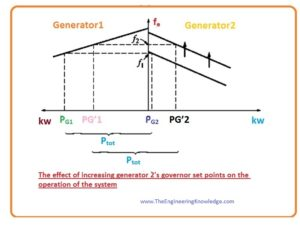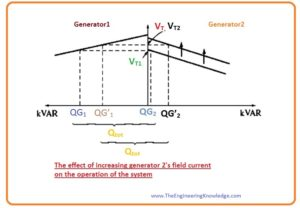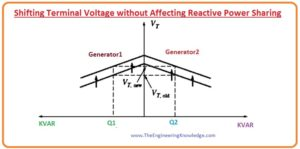Hello friends, I hope all of you are fine. In today’s tutorial, we are gonna have a look at Synchronous Generators Parallel with Same Size Generators. As we discussed in the synchronous generator operating alone  article that the when a single synchronous generator is working in system the active power (P) and reactive power (Q) provided by the generator both remains constant, constrained to be equivalent to the power required by the load, and the frequency  and output voltage VT were altered by the governor and the field current of the generator.

In the article Operation of Generators in Parallel with Large Power Systems, we also discussed that the frequency and output voltage was constrained to equal to the large power system, and the active P and reactive power Q were changed by the governor of the generator and the IF. In today’s post, we will discuss if we connect the generator parallel with another same size than what will be the effect on the terminal voltage, active power, and reactive power. So, let’s get started with the Operation of Generators in Parallel with Same Size Generators.

#### Synchronous Generators Parallel with Same Size Generators

• If the synchronous generator is attached in parallel with another synchronous generator that has equal power ratings. Then the resultant diagram of their connection is drawn in a given figure.• In this type of parallel operation, the summation of the active (P) and reactive power Q provided by these 2 generators should remain equal to the active and reactive power required by the load.
• In this parallel combination, the frequency of the system and the power of the synchronous generator should not be constant.
• The graphical representation among the frequency and the power is drawn in the given diagram, instantly when the second generator G2 is attached with the first.• The total power of this system is given as.

• The total reactive power Q of this system is given here.

Qtot =Qload = QG1 + QG2

What happens if the governor set points of G2 are increased?

• When the second’s generator governor setting points are increased, the curve among the power and frequency of the generator moves upward from its original point.• As we discussed earlier that the Ptot that is provided to the load should be constant.
• At the frequency f1 that is the original frequency of the system, the power provided by the generator G1 and G2 is higher than the requirements of the load, therefore this system will not work on the similar frequency as earlier.
• At the frequency f2, you can see in above-given figure that the total power of these two generators is identical to the load required power P The f2 is larger than the f1 original frequency of our system.
• At the frequency f2 the second generator providing larger power than earlier it was producing and the first generator now producing less power than before.
• If we summarize the effect of governor setting points increments on the parallel operation of the same size generator then we concluded that.
• The frequency of the system has increased as we can see that it increased from f1 to f2.
• By varying the governor points the generator’s (G2) power will increase and other (G1) generator’s power will decrease.
• Now we discuss the effect of field current of the generator G2 is varied.

What happens if the field current of G2 is increased?

• The resultant diagram when the field current of the G2 increased is drawn in a given figure.• In parallel operation of the 2-similar size generator when field current increase of second generator then we get these results.
• There will be an increment in the terminal voltage of both these 2 generators systems.
• The reactive power of the generator G2 has increased while the reactive power of the generator G1 has decreased.
• We noted that the in parallel operation of 2 similar size generator, the variation in the governor setting points of any generator will alter the overall frequency and power of both generators.

How can the power-sharing of the power system can adjust independently of the system frequency, and vice versa?

• The answer to this question is simplest. As we know that if governor setting points of any generator are increased then the frequency and the power of that generator, will also increase.
• And decrement in the set point of generator’s governor will decrease its power and the frequency.
• So, to regulate power-sharing without altering the system’s frequency, enhance the governor setting points of the first generator and concurrently lessen the governor’s setting points of the 2nd You can see this configuration in the given diagram.• Also, to regulate the system’s frequency without altering the power-sharing, concurrently do increment or decrement in the governor setting points. This arrangement also shown in given picture.• The regulation of the output voltage VT and reactive power can be done by this similar method. To move reactive power sharing points on the curve without varying the terminal voltage, concurrently upsurge the IF of first generator and do decrement in the field current of the second generator. It is shown in a given figure.• Correspondingly, to regulate the system’s frequency without altering the power-sharing, concurrently do increment or decrement in the governor setting points both the generators.#### Conclusion of the Operation of Generators in Parallel with Same Size Generators

• If we summarize the parallel operation of the same size generator than we get some main points that are described here.
• The sum of the power provided by the generator G1 and G2 should equal to the power used by the load.
• To regulate the active power distribution among both generators without altering system’s frequency concurrently increase the governor setting points on first generator and do decrement in the governor setting points of second generator. The increment in the governor setting points of that generator was done will take more load than others.
• To do adjustment in the frequency of the system without varying the active power-sharing, concurrently do increment or decrement in the governor setting points of these 2 generators.
• To regulate the reactive power (Q) distribution among these 2 generators without altering VT, concurrently do increment in the field current of one generator and decrease the field current of second generator. The generator will take more load whose field current has increased.
• To regulate VT without altering the reactive power (Q) distribution, concurrently do increment or decrement in the field current of both the generators.
• It is very significant that the synchronous generator operating in parallel with other generators shows a drooping characteristic among the frequency and the power.
• It is very important that any synchronous generator intended to operate in parallel with other machines have a drooping frequency-power characteristic.
• If 2 generators show the flat or almost flat characteristics, then the power distribution among them change extensively with the minor variation in the no-load speed.
• It shows in the given diagram.• You can see from the figure that the minor variation in the no-load speed of any generator would cause large changes in power-sharing.
• To maintain suitable power-sharing among both generators, their speed drop should be two to five %.

You can also read some related topics to synchronous generator that are listed here.

Introduction to Synchronous Generator

Synchronous Generator Equivalent Circuit

Synchronous Generator Phasor Diagram

Synchronous Generator Power and Torque

Synchronous Generator Parameters

Synchronous Generator Operating Alone

Synchronous Generator Parallel Operation

Synchronous Generator parallel with Large Power system

Synchronous Generator Ratings

Synchronous Generator Capability Curves

Synchronous Generator Transients

That’s all about Synchronous Generators Parallel with Same Size Generators I have mentioned each and everything related to Synchronous Generators Parallel with Same Size Generators. If you have any query ask in comments. See you in the next tutorial Synchronous Generator Ratings.

### 1 Comment

1. blog says:

Awesome! Its in fact amazing piece of writing, I have got